## RD Sharma Class 9 Solutions Chapter 21 Surface Areas and Volume of a Sphere Ex 21.1

These Solutions are part of RD Sharma Class 9 Solutions. Here we have given RD Sharma Class 9 Solutions Chapter 21 Surface Areas and Volume of a Sphere Ex 21.1

Other Exercises

Question 1.
Find the surface area of a sphere of radius.
(i) 10.5 cm
(ii) 5.6 cm
(iii) 14 cm
Solution:
In a sphere,
(i) Radius (r) = 10.5 cm
Surface area = 4πr2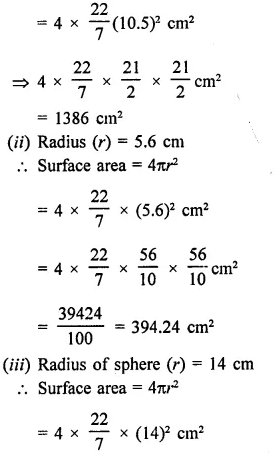Question 2.
Find the surface area of a sphere of diameter
(i) 14 cm
(ii) 21 cm
(iii) 3.5 cm
Solution:
(i) Diameter of a sphere = 14 cm
Radius (r) = $$\frac { 14 }{ 2 }$$ = 7 cmQuestion 3.
Find the total surface area of a hemisphere and a solid hemisphere each of radius 10 cm. [Use π = 3.14]
Solution:
(i) Radius of hemisphere = 10 cm
∴ Total surface area of hemisphere = 2πr2
= 2 x 3.14 x 10 x 10 cm2
= 628 cm2
(ii) Total surface area of solid hemisphere
= 3πr2 = 3 x 3.14 x 10 x 10 cm2
= 942 cm2

Question 4.
The surface area of a sphere in 5544 cm2, find the diameter.
Solution:
Let r be the radius of a sphere, then Surface area = 4πr2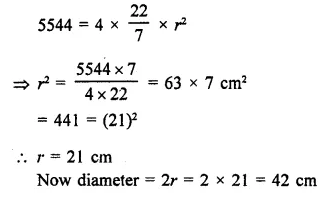Question 5.
A hemispherical bowl made of brass has inner diameter 10.5 cm. Find the cost of tin-plating it on the inside at the rate of ₹4 per 100 cm2. [NCERT]
Solution:
Inner diameter of a hemispherical bowl = 10.5 cm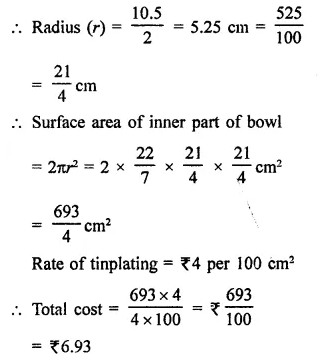Question 6.
The dome of a building is in the form of a hemisphere. Its radius is 63 dm. Find the cost of painting it at the rate of ₹2 per sq. m.
Solution:
Radius of dome (hemispherical) = 63 dm
Area of curved surface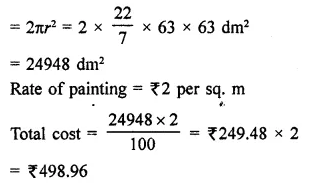Question 7.
Assuming the earth to be a sphere of radius 6370 km, how many square kilometres is area of the land, if three-fourth of the earth’s surface is covered by water?
Solution:
Radius of earth (sphere) = 6370 km
Water on the earth = $$\frac { 3 }{ 4 }$$ % total area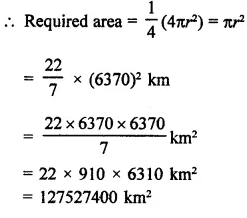Question 8.
A cylinder of same height and radius is placed on the top of a hemisphere. Find the curved surface area of the shape if the length of the shape be 7 cm.
Solution:
Total height of the so formed shape = 7 cm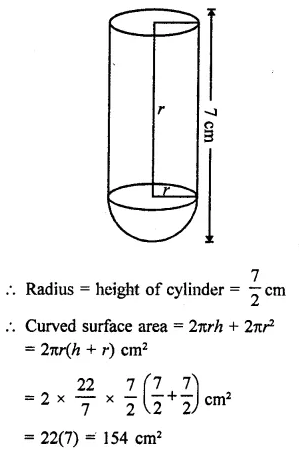Question 9.
The diameter of the moon is approximately one fourth of the diameter of the earth. Find the ratio of their surface areas.
Solution:
Diameter of moon = $$\frac { 1 }{ 4 }$$ of diameter of earth
Let radius of earth = r km
Then radius of moon = $$\frac { 1 }{ 4 }$$ r km
Now surface area of earth = 4πr2Question 10.
A hemi-spherical dome of a building needs to be painted. If the circumference of the base of the dome is 17.6 m, find the cost of painting it, given the cost of painting is ₹5 per 100 cm2. [NCERT]
Solution:
Circumference of the base of dome (r) = 17.6 m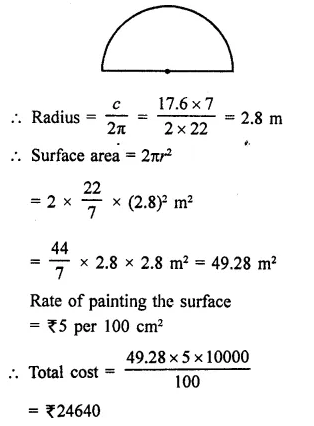Question 11.
A wooden toy is in the form of a cone surmounted on a hemisphere. The diameter of the base of the cone is 16 cm and its height is 15 cm. Find the cost of painting the toy at ₹7 per 100 cm2.
Solution:
Diameter of toy = 16 cm
Radius (r) = $$\frac { 16 }{ 2 }$$ = 8 cm
Height of conical part (h) = 15 cm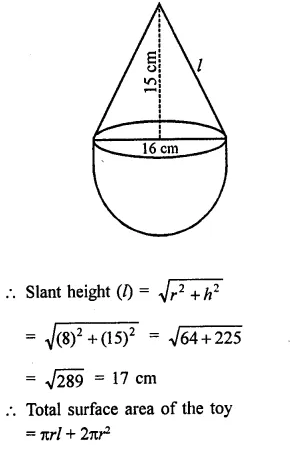Question 12.
A storage tank consists of a circular cylinder with a hemisphere adjoined on either end. If the external diameter of the cylinder be 1.4 m and its length be 8 m, find the cost of painting it on the outside at the rate of ₹10 per m2.
Solution:
Diameter of the tank = 1.4 m
∴ Radius (r) = $$\frac { 1.4 }{ 2 }$$ m = 0.7 m
and height of cylindrical portion = 8m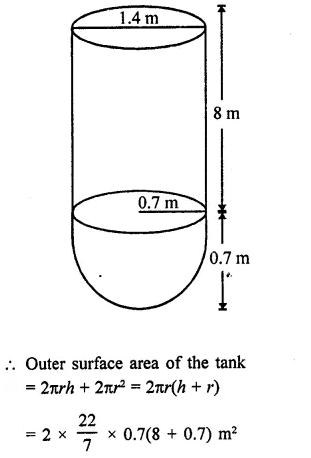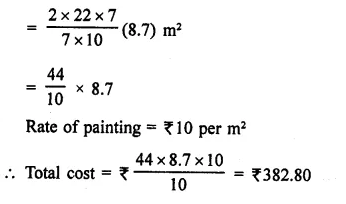Question 13.
The front compound wall of a house is decorated by wooden spheres of diameter 21 cm, placed on small supports as shown in the figure. Eight such spheres are used for this purpose, and are to be painted silver. Each support is a cylinder of radius 1.5 cm and height 7 cm and is to be painted black. Find the cost of paint required if silver paint costs 25 paise per cm2 and black paint costs 5 paise per cm2. [NCERT]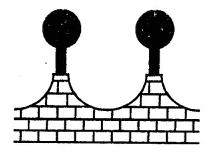Solution:
Diameter of each spheres = 21 cm
∴ Radius (R) = $$\frac { 21 }{ 2 }$$ cm
Radius of each cylinder (r) = 1.5 cm
and height (h) = 7 cm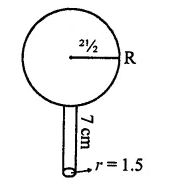Now surface area of one sphere = 4πR2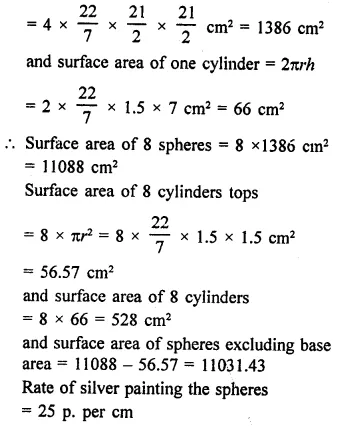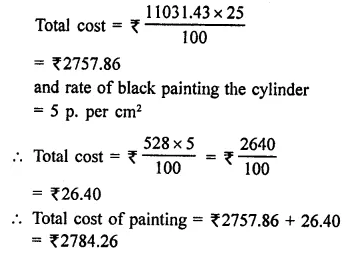Hope given RD Sharma Class 9 Solutions Chapter 21 Surface Areas and Volume of a Sphere Ex 21.1 are helpful to complete your math homework.

If you have any doubts, please comment below. Learn Insta try to provide online math tutoring for you.